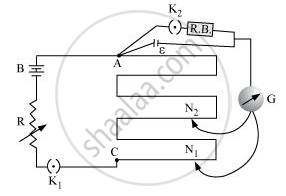PUC Karnataka Science Class 12Department of Pre-University Education, Karnataka
Share

# Write the Principle of Working of a Potentiometer. Describe Briefly, with the Help of a Circuit Diagram, How a Potentiometer is Used to Determine the Internal Resistance of a Given Cell. - PUC Karnataka Science Class 12 - Physics

#### Question

Write the principle of working of a potentiometer. Describe briefly, with the help of a circuit diagram, how a potentiometer is used to determine the internal resistance of a given cell.

#### Solution

The working principle of a potentiometer is based on Kirchhoff’s voltage law. According to this rule, the algebraic sum of changes in voltage around any closed loop involving resistors and cells in the loop is zero.Let Φ be the potential drop per unit length in the potentiometer wire.

When only a cell is connected, the balance point is N1.

Appling Kirchhoff’s voltage law,

ε = Φ l1 [l1 = Length at which the balance point is achieved]

When some current is drawn using the resistance box, the balance point is achieved at N2.

V = Φl2

This gives,

epsi/V = l_1 / l_2

ε = I (r + R) [R = Resistance of the resistance box]

V = IR

This gives,

epsi /V = (r+R)/R

r = R (l_1/l_2  - 1)

The internal resistance of the cell can be determined by plugging-in the measured values of l1 and l2.

Is there an error in this question or solution?

#### APPEARS IN

Solution Write the Principle of Working of a Potentiometer. Describe Briefly, with the Help of a Circuit Diagram, How a Potentiometer is Used to Determine the Internal Resistance of a Given Cell. Concept: Potentiometer.
S